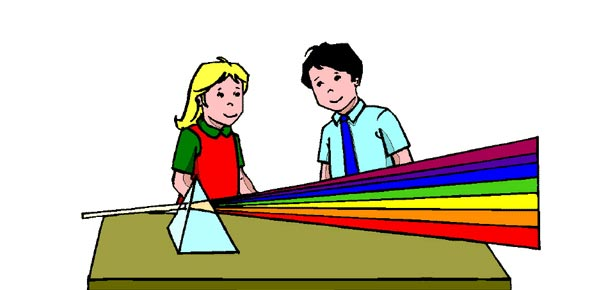# Lighting: Stage Tech 1

34 Questions | Total Attempts: 170SettingsIn any stage set up, we have different sections of concern, which require different forms of expertise. Lighting is one of the essential ones and the lighting: stage tech 1 quiz below has more on this. All the best.

• 1.
What are the three types of lights?
• A.

Ellipsoidal

• B.

Parabolic

• C.

Concave

• D.

Spherical

• 2.
What is a lens system?
• 3.
What is Ohms?
• A.

Work

• B.

Resistance

• C.

Current

• D.

Potential pressure

• 4.
What is wattage?
• A.

Work

• B.

Current

• C.

Potential pressure

• D.

Resistance

• 5.
What is amps?
• A.

Potential pressure

• B.

Work

• C.

Current

• D.

Resistance

• 6.
What is voltage?
• A.

Potential pressure

• B.

Work

• C.

Resistance

• D.

Current

• 7.
What is an alternating current?
• A.

One that moves back and forth

• B.

A change in current

• C.

Moves from positive to negative

• D.

Moves negative to positive

• 8.
What is a direct current?
• A.

One that moves negative to positive

• B.

One that moves positive to negative

• C.

One that hits the direct source of power

• D.

One that goes back and forth

• 9.
What is the state used to remember the W/v a formula?
• 10.
Find Watts:3-12 amps
• 11.
Find watts:4-8 amps
• 12.
Find watts:7-6 amps
• 13.
Find amperage:5-150watts
• 14.
Find amperage:7-120watts
• 15.
Find Amperage 4-30watts
• 16.
What is a cut list?
• A.

A lis of the materials you cut from using the show

• B.

A list of every gel to be used on every fixture

• C.

A list of measurements on how to cut gels

• D.

A list of cuts you got during your show process

• 17.
An instrument schedule is a form used to record all of the technical data about each instrument used in the production, also known as a hookup sheet.
• A.

True

• B.

False

• 18.
What does a dimmer do?
• A.

Dims the lights

• B.

A device used to control the amount of light

• C.

A device that stops light

• D.

A device that gives light

• 19.
First electric is a pipe that hangs off stage
• A.

True

• B.

False

• 20.
Which of the following is a gate accessory?
• A.

Yolk

• B.

Barn doors

• C.

Dimmer

• D.

Gobo

• 21.
What is a beam designation used with?
• A.

Gobo's

• B.

srs's spherical reflector spots

• C.

Ers's ellipsoidal reflector spot

• D.

prs's parabolic reflector spot

• 22.
What is a color media
• A.

Color wheel

• B.

Gel

• C.

Cyan

• D.

Magenta

• 23.
What is a focus area?
• A.

Area where the light should be focused, that will correspond with the same letter identifies a specific lighting area on the plot

• B.

Area where light should be focused

• C.

Area that wants to be focused and isn't

• D.

Area that doesn't care if it is focused or not

• 24.
Hanging cards are cards that you hang from the celing with numbers on them that determine the position of the lighting instrument/
• A.

True

• B.

False

• 25.
What is a channel?
• A.

A channel is something you watch on tv, usually disney channel

• B.

Shows the assigned channel for the instrument( how light board controls light)

• C.

The button you press to make the lights change

• D.

A electrical outlet that is numbered to know what light should be plugged in where

Related TopicsBack to top